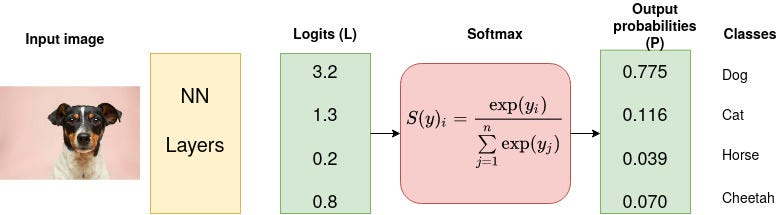# Softmax

It is often used as the last activation function of a neural network to normalize the output of a network to a probability distribution over predicted output classes.
Softmax is an activation function that scales numbers/logits into probabilities. The output of a Softmax is a vector (say v) with probabilities of each possible outcome. The probabilities in vector v sums to one for all possible outcomes or classes.
Mathematically, Softmax is defined as,
$S(y)_i = \frac{exp(y_i)}{\sum_{j=1}^n exp(y_j)}$

### Example

Consider a CNN model which aims at classifying an image as either a dog, cat, horse or cheetah (4 possible outcomes/classes). The last (fully-connected) layer of the CNN outputs a vector of logits, L, that is passed through a Softmax layer that transforms the logits into probabilities, P. These probabilities are the model predictions for each of the 4 classes.Image for post
Let us calculate the probability generated by the first logit after Softmax is applied,
$exp(3.2)=24.5325, exp(1.3) = 3.6693, exp(0.2)=1.2214, exp(0.8)=2.2255$
Therefore,
$S(3.2) = \frac{exp(3.2)}{exp(3.2)+exp(1.3)+exp(0.2)+exp(0.8)} = 0.775$
And similar for softmax of 1.3, 0.2, and 0.8